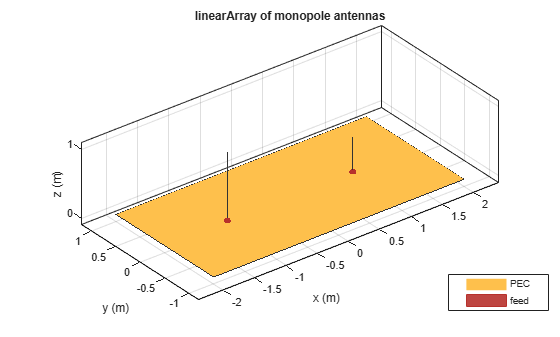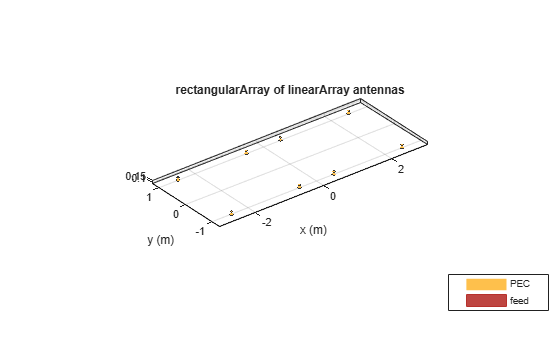# linearArray

Create linear antenna array

## Description

The `linearArray` object creates a linear antenna array in the xy-plane. The default linear array is a two-element dipole array. The dipoles are center-fed. Each dipole resonates at 70MHz when isolated.## Creation

### Syntax

``array = linearArray``
``array = linearArray(Name=Value)``

### Description

example

``` `array = linearArray` creates a linear antenna array in the X-Y plane. ```

example

``` `array = linearArray(Name=Value)` creates a linear antenna array with additional Properties specified by one or more name-value arguments. `Name` is the property name and `Value` is the corresponding value. You can specify several name-value pair arguments in any order as `Name1=Value1`, `...`, `NameN=ValueN`. Properties that you do not specify retain their default values.For example, `linArr = linearArray(Element=discone)` creates a linear array of two discone antennas.```

### Output Arguments

expand all

Linear array, returned as an `linearArray` object.

## Properties

expand all

Individual antenna type, specified as a single antenna object for homogeneous array or a vector of antenna objects of same type with varying dimensions and load for heterogeneous array. The antenna object can be an element from the Antenna Catalog, or a `linearArray`, or an antenna without a substrate created using `customAntennaGeometry`, `customAntennaMesh`, `customAntennaStl`, or `customAntenna` objects.

Note

Following antennas are unsupported as an element:

Example: `monopole`

Example: `[monopole monopole(Height=2)]`

Example: `linearArray`

Example: `customAntenna`

Number of antenna or linear array elements in array, specified as a scalar.

Example: 4

Spacing between antenna elements or arrays in array, specified as a scalar or vector in meters. By default, the dipole elements are spaced 2m apart.

Example: `3`

Data Types: `double`

Excitation amplitude of antenna elements, specified as a scalar or vector. Set the property value to `0 ` to model dead elements. This value corresponds to the excitation voltages for the elements in the array.

Example: `3`

Data Types: `double`

Phase shift for antenna elements, specified as a scalar or vector in degrees. This value corresponds to the excitation voltages for the elements in the array.

Example: `[3 3 0 0]`

Data Types: `double`

Tilt angle of the array in degrees, specified as a scalar or vector. For more information, see Rotate Antennas and Arrays.

Example: `90`

Example: `Tilt=[90 90]`,`TiltAxis=[0 1 0;0 1 1]` tilts the array at 90 degrees about the two axes, defined by the vectors.

Data Types: `double`

Tilt axis of the array, specified as one of these values:

• Three-element vectors of Cartesian coordinates in meters. In this case, each vector starts at the origin and lies along the specified points on the x-, y-, and z-axes.

• Two points in space, specified as a 2-by-3 matrix corresponding to two three-element vectors of Cartesian coordinates. In this case, the array rotates around the line joining the two points.

• `"x"`, `"y"`, or `"z"` to describe a rotation about the x-, y-, or z-axis, respectively.

Example: `[0 1 0]`

Example: `[0 0 0;0 1 0]`

Example: `"Z"`

Data Types: `double` | `string`

## Object Functions

 `arrayFactor` Array factor in dB `beamwidth` Beamwidth of antenna `charge` Charge distribution on antenna or array surface `correlation` Correlation coefficient between two antennas in array `current` Current distribution on antenna or array surface `design` Design prototype antenna or arrays for resonance around specified frequency or create AI-based antenna from antenna catalog objects `efficiency` Radiation efficiency of antenna `EHfields` Electric and magnetic fields of antennas or embedded electric and magnetic fields of antenna element in arrays `impedance` Input impedance of antenna or scan impedance of array `info` Display information about antenna or array `layout` Display array or PCB stack layout `mesh` Mesh properties of metal, dielectric antenna, or array structure `optimize` Optimize antenna or array using SADEA optimizer `pattern` Plot radiation pattern and phase of antenna or array or embedded pattern of antenna element in array `patternAzimuth` Azimuth plane radiation pattern of antenna or array `patternElevation` Elevation plane radiation pattern of antenna or array `rcs` Calculate and plot radar cross section (RCS) of platform, antenna, or array `returnLoss` Return loss of antenna or scan return loss of array `show` Display antenna, array structures, shapes, or platform `sparameters` Calculate S-parameters for antennas and antenna arrays `vswr` Voltage standing wave ratio (VSWR) of antenna or array element

## Examples

collapse all

Create a linear array of four dipoles and plot the layout of the array.

```la = linearArray; la.NumElements = 4; layout(la);```Plot the radiation pattern of a four element linear array of dipoles at a frequency 70MHz.

```la = linearArray(NumElements=4); pattern(la,70e6);```Create a linear array of two monopoles.

```m1 = monopole; m2 = monopole(Height=0.5); mla = linearArray```
```mla = linearArray with properties: Element: [1x1 dipole] NumElements: 2 ElementSpacing: 2 AmplitudeTaper: 1 PhaseShift: 0 Tilt: 0 TiltAxis: [1 0 0] ```
```mla.Element = [m1 m2]; show(mla);```Create an array of discones with 3 m. element spacing.

```la = linearArray(Element=discone); la.ElementSpacing = 3; show(la)```Create a rectangular array of the linear array.

`ra = rectangularArray(Element=la)`
```ra = rectangularArray with properties: Element: [1x1 linearArray] Size: [2 2] RowSpacing: 2 ColumnSpacing: 2 Lattice: 'Rectangular' AmplitudeTaper: 1 PhaseShift: 0 Tilt: 0 TiltAxis: [1 0 0] ```
`show(ra)`Create a linear array of a linear array with 1 m. element spacing and plot the directivity pattern at 70 MHz.

```la = linearArray(Element=linearArray(ElementSpacing=1)); show(la)````pattern(la,70e6); `Balanis, C.A. Antenna Theory. Analysis and Design, 3rd Ed. New York: Wiley, 2005.